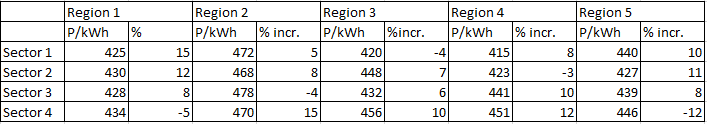### CAT 1997 Question Paper Question 18

Instructions

The following table gives the tariff [in paise per kilo-watt-hour (kWh)] levied by the UPSEB in 1994-95, in four sectors and the regions within them. The table also gives the percentage change in the tariff as compared to 1991-92.Question 18

# If the amount of power consumed by the various regions in sector 1 is the same, then as compared to 1991-92 the net tariff in 1994-95

Solution

Average tariff in Sector 1 in 1994-95 = $$\frac{425 + 472 + 420 + 415 + 440}{5}$$ = 434.4 P/kWh

Average tariff in Sector 1 in 1991-92=$$\frac{\frac{425}{1.15}+\frac{472}{1.05}+\frac{420}{0.96}+\frac{415}{1.08}+\frac{440}{1.1}}{5}$$=408.4 P/kWh

Change =434.4-408.4=26 P/kWh

% Change = $$\frac{26}{408.4}\times 100$$ = 6.5%

• All Quant CAT Formulas and shortcuts PDF
• 30+ CAT previous papers with solutions PDF